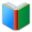Words for students of 7th Grade, the page is for math words (List 1), which are essential vocabulary for 7th Grade academic classes.
 Actions upon current list

 All lists of current grdae
Spelling Words:

Arts Words:

Social Words:

Math Words:

Science Words:

Literature Words:The Shoes of FortuneThe ShadowThe Adventures of Sherlock HolmesAdventures of Huckleberry Finn
 7th Grade Cards: Math (click the card to toggle it.)
a real number regardless of its sign
a triangle whose interior angles are all acute
the line that divides something into two equal parts
when two lines are crossed by another line, the angles in matching corners
give reduction in price on; an amount or percentage deducted
a three-sided regular polygon
the ratio of the number of outcomes in which a specified event occurs to the total number of trials, not in a theoretical sample space but in an actual experiment.
scientific notation, a way of writing numbers that are too big or too small to be conveniently written in decimal form
the angle between a side of a rectilinear figure and an adjacent side extended outward
the power of attracting or a fixed charge for borrowing money; usually a percentage of the amount borrowed
the angle between adjacent sides of a rectilinear figure
a triangle with two equal sides
less than zero; having a negative charge; reckoned in a direction opposite to that regarded as positive
a triangle that contains an obtuse interior angle
result; end or consequence; result of a random trial
rearrangement of the elements of a set
involving advantage or good ; greater than zero; very sure; confident